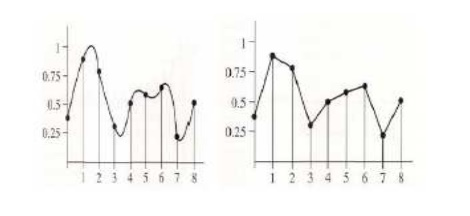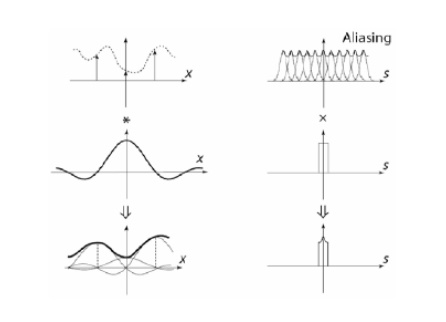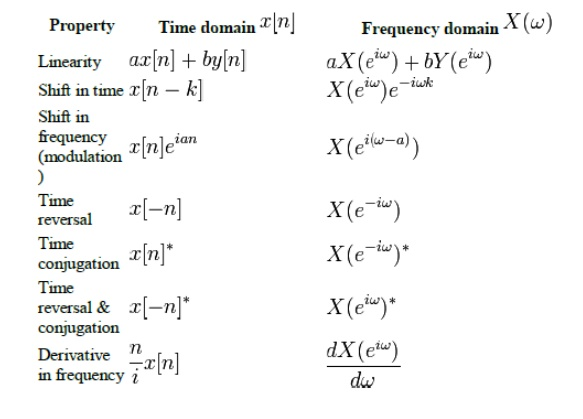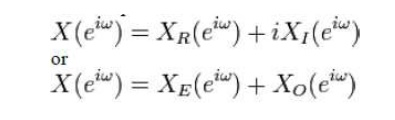Home | | Signals and Systems | Analysis of Discrete Time Signals

# Analysis of Discrete Time Signals

Let x(t) be a continuous signal which is to be sampled, and that sampling is performed by measuring the value of the continuous signal every T seconds, which is called the sampling interval.

ANALYSIS OF DISCRETE TIME SIGNALS

Sampling theory

Let x(t) be a continuous signal which is to be sampled, and that sampling is performed by measuring the value of the continuous signal every T seconds, which is called the sampling interval. Thus, the sampled signal x[n] given by:x[n] = x(nT), with n = 0, 1, 2, 3, ...

The sampling frequency or sampling rate fs is defined as the number of samples obtained in one second, or fs = 1/T. The sampling rate is measured in hertz or in samples per second.

The frequency equal to one-half of the sampling rate is therefore a bound on the highest frequency that can be unambiguously represented by the sampled signal. This frequency (half the sampling rate) is called the Nyquist frequency of the sampling system. Frequencies above the Nyquist frequency fN can be observed in the sampled signal, but their frequency is ambiguous. That is, a frequency component with frequency f cannot be distinguished from other components with frequencies NfN + f and NfNf for nonzero integers N. This ambiguity is called aliasing. To handle this problem as gracefully as possible, most analog signals are filtered with an anti-aliasing filter (usually a low-pass filter with cutoff near the Nyquist frequency) before conversion to the sampled discrete representation.

The theory of taking discrete sample values (grid of color pixels) from functions defined over continuous domains (incident radiance defined over the film plane) and then using those samples to reconstruct new functions that are similar to the original (reconstruction).

Sampler: selects sample points on the image plane

Filter: blends multiple samples togetherSampling Theorem:

Sampling Theorem: bandlimited signal can be reconstructed exactly if it is sampled at a rate atleast twice the maximum frequency component in it."

Consider a signal g(t) that is bandlimited.The maximum frequency component of g(t) is fm. To recover the signal g(t) exactly from its samples it has to be sampled ata rate fs _ 2fm. The minimum required sampling rate fs = 2fm is called nyquist rate

A continuous time signal can be processed by processing its samples through a discrete time system. For reconstructing the continuous time signal from its discrete time samples without any error, the signal should be sampled at a sufficient rate that is determined by the sampling theorem.

Aliasing

Aliasing is a phenomenon where the high frequency components of the sampled signal interfere with each other because of inadequate sampling ωs< 2ωm. AliasingAliasing leads to distortion in recovered signal. This is the reason why sampling frequency should be atleast twice the bandwidth of the signal.

Sampling of Non-bandlimited Signal: Anti-aliasing Filter

Anti aliasing filter is a filter which is used before a signal sampler, to restrict the

bandwidth of a signal to approximately satisfy the sampling theorem. The potential defectors are all the frequency components beyond fs/2 Hz. We should have to eliminate these components from x(t) before sampling x(t). As a result of this we lose only the components beyond the folding frequency fs/2 Hz. These frequency components cannot reappear to corrupt the components with frequencies below the folding frequency. This suppression of higher frequencies can be accomplished by an ideal filter of bandwidth fs/2 Hz. This filter is called the anti-aliasing filter. The anti aliasing operation must be performed before the signal is sampled. The anti aliasing filter, being an ideal filter is unrealizable. In practice, we use a steep cutoff filter, which leaves a sharply attenuated residual spectrum beyond the folding frequency fs/2.

DISCRETE TIME FOURIER TRANSFORM

In mathematics, the discrete-time Fourier transform (DTFT) is one of the specific

forms of Fourier analysis. As such, it transforms one function into another, which is called the frequency domain representation, or simply the "DTFT", of the original function (which is often a function in the time-domain). But the DTFT requires an input function that is discrete. Such inputs are often created by sampling a continuous function, like a person's voice.Inverse transform

The following inverse transforms recover the discrete-time sequence:The integrals span one full period of the DTFT, which means that the x[n] samples are also the coefficients of a Fourier series expansion of the DTFT.

Infinite limits of integration change the transform into a continuous-time Fourier transform [inverse], which produces a sequence of Dirac impulses. That is:PropertiesSYMMETRY PROPERTIES

The Fourier Transform can be decomposed into a real and imaginary part or into an even and odd part.Z-transforms

Definition: The Z – transform of a discrete-time signal  x(n) is defined as the power series:where z is a complex variable. The above given relations are sometimes called the direct Z-transform because they transform the time-domain signal x(n)into its complex-plane representation X(z). Since Z – transform is an infinite power series, it exists only for those values of z for which this series converges.

The region of convergence of X(z) is the set of all values of z for which X(z) attains a finite value.

For discrete-time systems, z-transforms play the same role of Laplace transforms do in continuous-time systemsUsing the definition requires a contour integration in the complex z-plane.

Fortunately, we tend to be interested in only a few basic signals (pulse, step, etc.) Virtually all of the signals we’ll see can be built up from these basic signals.

Z transform propertiesStudy Material, Lecturing Notes, Assignment, Reference, Wiki description explanation, brief detail
Signals and Systems : Analysis of Discrete Time Signals : Analysis of Discrete Time Signals |

Related Topics